CLAT  >  Test: Quantitative Techniques

# Test: Quantitative Techniques

Test Description

## 20 Questions MCQ Test Additional Study Material for CLAT | Test: Quantitative Techniques

Test: Quantitative Techniques for CLAT 2023 is part of Additional Study Material for CLAT preparation. The Test: Quantitative Techniques questions and answers have been prepared according to the CLAT exam syllabus.The Test: Quantitative Techniques MCQs are made for CLAT 2023 Exam. Find important definitions, questions, notes, meanings, examples, exercises, MCQs and online tests for Test: Quantitative Techniques below.
Solutions of Test: Quantitative Techniques questions in English are available as part of our Additional Study Material for CLAT for CLAT & Test: Quantitative Techniques solutions in Hindi for Additional Study Material for CLAT course. Download more important topics, notes, lectures and mock test series for CLAT Exam by signing up for free. Attempt Test: Quantitative Techniques | 20 questions in 15 minutes | Mock test for CLAT preparation | Free important questions MCQ to study Additional Study Material for CLAT for CLAT Exam | Download free PDF with solutions
 1 Crore+ students have signed up on EduRev. Have you?
Test: Quantitative Techniques - Question 1

### How much above the cost price should a man mark his goods, so that, after allowing a discount of 10% for cash payment, he may still make a profit of 8%?

Detailed Solution for Test: Quantitative Techniques - Question 1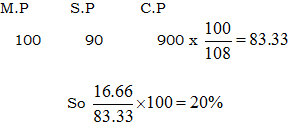Test: Quantitative Techniques - Question 2

### Two numbers are respectively 20% and 50% more than a third number. These two numbers are in the ratio:

Detailed Solution for Test: Quantitative Techniques - Question 2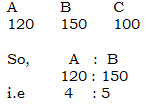Test: Quantitative Techniques - Question 3

### If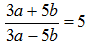, then a : b is equal to:

Detailed Solution for Test: Quantitative Techniques - Question 3

Go via options (d) option satisfies it.

Test: Quantitative Techniques - Question 4

The ratio of the present ages of puneet and Appu is 2 : 3. After 3 years the ratio of their ages will be 3 : 4. The present age of Puneet is:

Detailed Solution for Test: Quantitative Techniques - Question 4

Ratio (1) = 3 yrs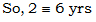Test: Quantitative Techniques - Question 5

Annual incomes of A and B are in the ratio 4 : 3 and their annual expenses are in the ratio 3 : 2. If each of them saves Rs. 600 at the end of the year, what is the annual income of A?

Detailed Solution for Test: Quantitative Techniques - Question 5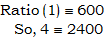Test: Quantitative Techniques - Question 6

The average of marks in Mathematics for 5 students was found to be 50. Later, it was discovered that in the case of one student the marks 48 were misread as 84. The correct average is:

Detailed Solution for Test: Quantitative Techniques - Question 6

Out – 84
In  - 48
Diff = 36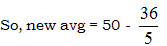= 42.8

Test: Quantitative Techniques - Question 7

If the cost price of 10 articles is equal to the selling price of 7 articles, then the gain or loss per cent is:

Detailed Solution for Test: Quantitative Techniques - Question 7

C.P (10) = S.P (7)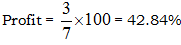Test: Quantitative Techniques - Question 8

Two bicycles were sold for Rs. 3990 each, gaining 5% on one and losing 5% on other. The gain or loss per cent on the whole transaction is:

Detailed Solution for Test: Quantitative Techniques - Question 8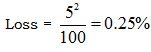Test: Quantitative Techniques - Question 9

Two trains are running with speeds 30 km/hr and 58 km/hr in the same direction. A man in the slower train passes the faster train in 18 seconds. The length (in metres) of the faster train is:

Detailed Solution for Test: Quantitative Techniques - Question 9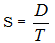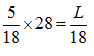So, L = 140 m

Test: Quantitative Techniques - Question 10

In a school 40% of the students play football and 50% play cricket. If 18% of the students neither play football nor cricket, the percentage of the students playing both is:

Detailed Solution for Test: Quantitative Techniques - Question 10

F + C = 90%
But, 100% - 18% = 82%
So, Diff = 90% - 82% = 8%

Test: Quantitative Techniques - Question 11

If the side of a square is increased by 25%, then its area is increased by:

Detailed Solution for Test: Quantitative Techniques - Question 11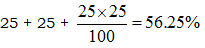Test: Quantitative Techniques - Question 12

A student has to secure 40% marks to pass. He gets 90 marks and fails by 10 marks. Maximum marks are:

Detailed Solution for Test: Quantitative Techniques - Question 12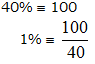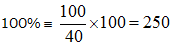Test: Quantitative Techniques - Question 13

If A’s height is 10% more than B’s height, by how much per cent less is B’s height than that of A?

Detailed Solution for Test: Quantitative Techniques - Question 13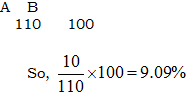Test: Quantitative Techniques - Question 14

A reduction of 20% in the price of sugar enables a purchaser to obtain 3 kg more for Rs. 120. The original price (per kg) of sugar is:

Detailed Solution for Test: Quantitative Techniques - Question 14

Reduction = 20%
Increase in quantity = 1/4
So 1/4 = 3 kg
So, original price = 120/12 = 10

Test: Quantitative Techniques - Question 15

If the difference between the simple and compound interests on a sum of money for 2 years at 4% per annum is Rs. 80, the sum is:

Detailed Solution for Test: Quantitative Techniques - Question 15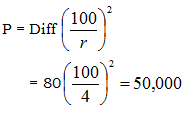Test: Quantitative Techniques - Question 16

A number, when divided by 119, leaves a remainder of 19. If it is divided by 17, it will leave a remainder of:

Detailed Solution for Test: Quantitative Techniques - Question 16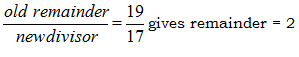Test: Quantitative Techniques - Question 17

The L.C.M. of two numbers is 20 times their H.C.F. The sum of H.C.F. and L.C.M. is 2520. If one of the numbers is 480, the other number is:

Detailed Solution for Test: Quantitative Techniques - Question 17

L x H = a x b
20 H x H = 480 x b
L + H = 2520
So, b = 600

Test: Quantitative Techniques - Question 18

The number, whose square is equal to the difference between the squares of 975 and 585, is:

Detailed Solution for Test: Quantitative Techniques - Question 18

x2 = (975)2 – (585)2
x2 = 608400
So, x = 780

Test: Quantitative Techniques - Question 19

A tap can fill a cistern in 8 hours and another tap can empty it in 16 hours. If both the taps are open, the time (in hours) taken to fill the tank will be:

Detailed Solution for Test: Quantitative Techniques - Question 19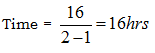Test: Quantitative Techniques - Question 20

Out of 10 teachers of a school, one teachers retires and in his place a new teacher of age 25 years joins. As a result of it, the average age of the teachers is reduced by 3 years. The age of the retired teacher is

Detailed Solution for Test: Quantitative Techniques - Question 20

Age = 25 + 3(10)
= 55 years

## Additional Study Material for CLAT

1 videos|19 docs|122 tests
 Use Code STAYHOME200 and get INR 200 additional OFF Use Coupon Code
Information about Test: Quantitative Techniques Page
In this test you can find the Exam questions for Test: Quantitative Techniques solved & explained in the simplest way possible. Besides giving Questions and answers for Test: Quantitative Techniques, EduRev gives you an ample number of Online tests for practice

## Additional Study Material for CLAT

1 videos|19 docs|122 tests